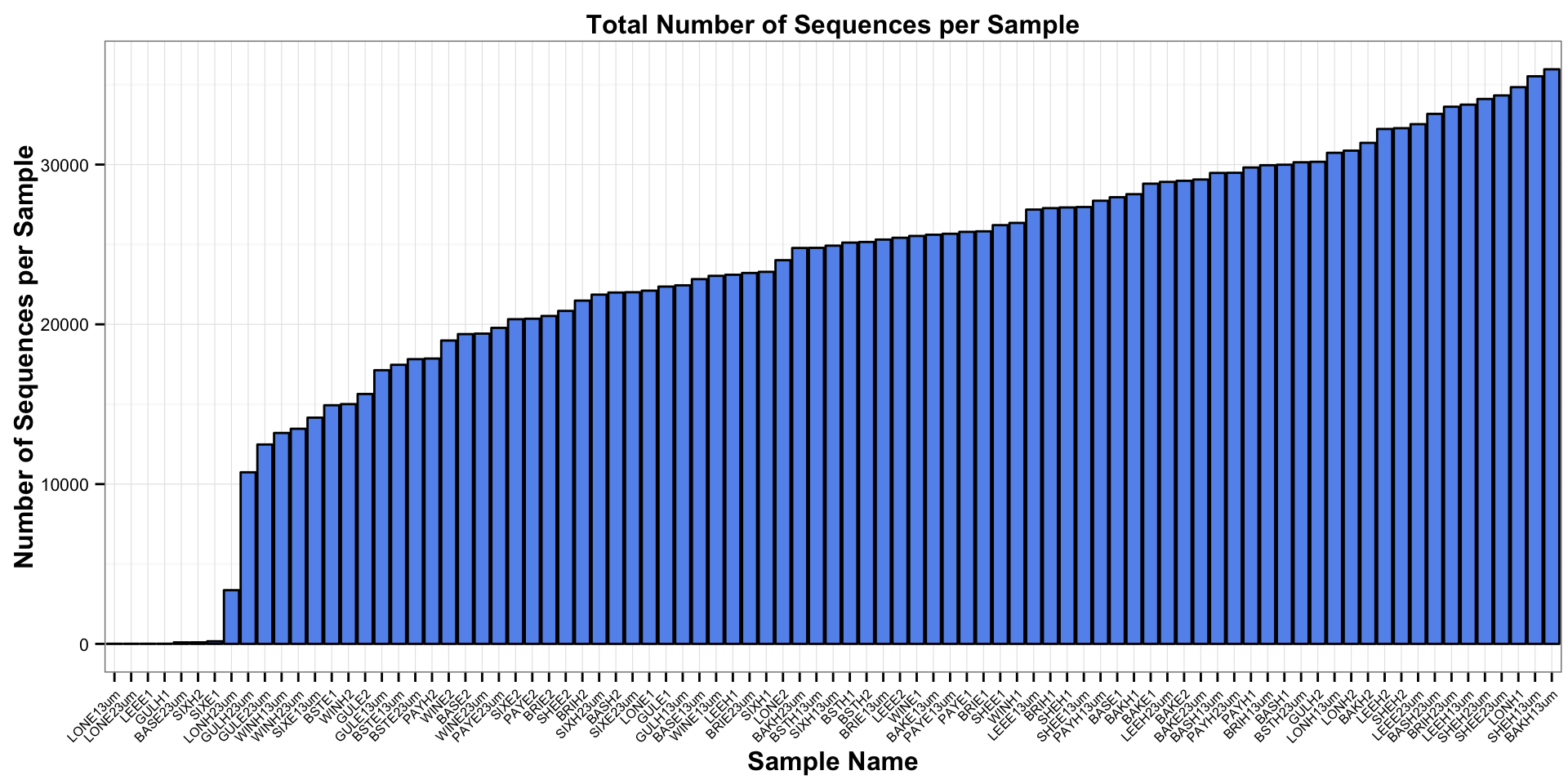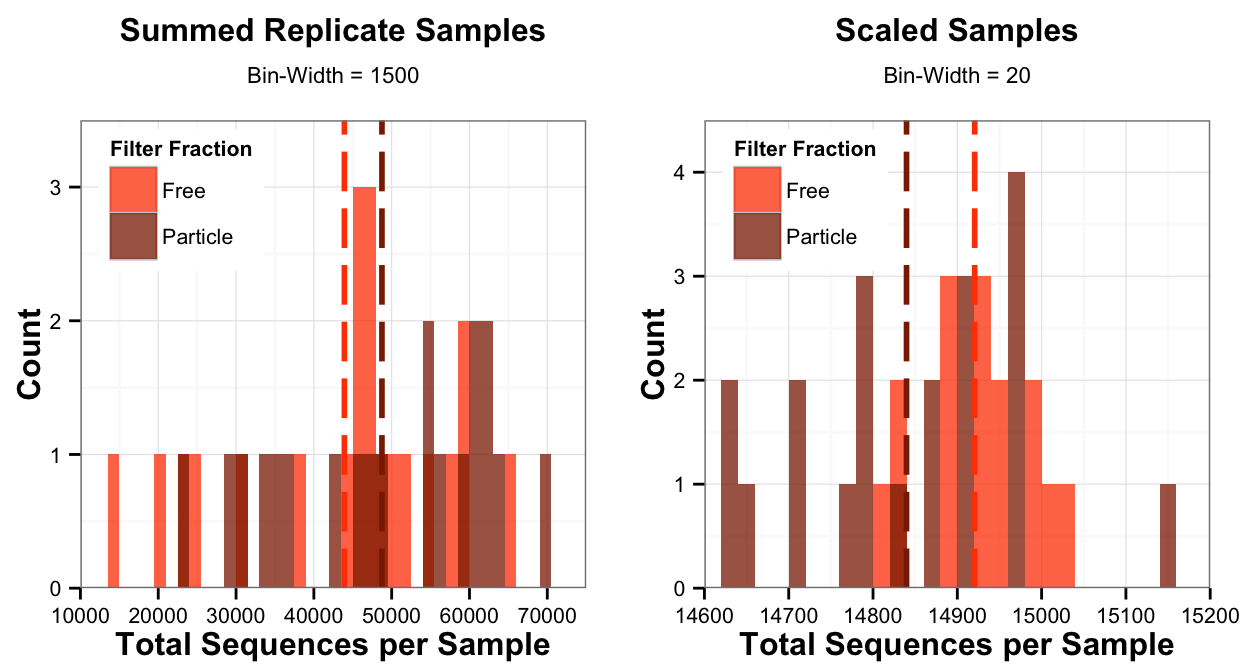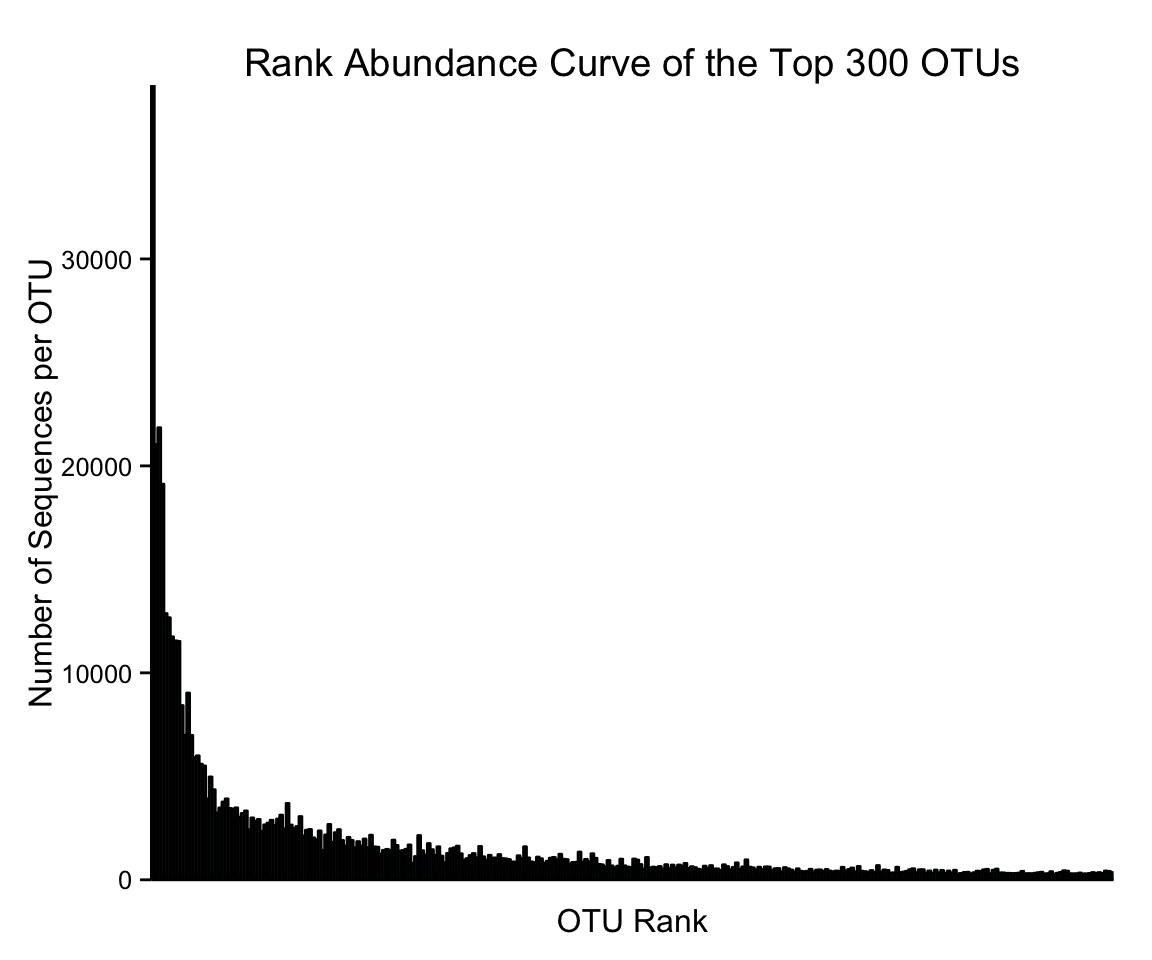### Set the Global Knitr Options

``````# Set the global options
knitr::opts_chunk\$set(echo= TRUE, eval = TRUE, fig.width=6, fig.height=5, fig.align = 'center',
warning=FALSE, message=FALSE)
# Set the seed
set.seed(15879966)``````

### Load in R-Packages and Set the Working Environment

``````# Load packages
necessary_packages <-  c("phyloseq", "ggplot2", "ape", "vegan", "plyr", "scales", "grid", "reshape2",
"data.table","DESeq2", "dplyr","tidyr", "sciplot","scales", "gtable","pgirmess",
"multcompView", "pander")

# Install the necessary packages
packages <- lapply(necessary_packages, library, character.only = TRUE)

# Set the working Directory
setwd("~/Final_PAFL_Trophicstate")

### Source written functions in the file Functions_PAFL.R that is housed within the "Final_PAFL_Trophicstate"
source("Functions_PAFL.R")``````

### Set the Plotting and Table Options

``````### Set our theme for our plots down the road
theme_set(theme_bw() + theme(plot.title = element_text(face="bold", size = 12),  #Set the plot title
strip.text.x = element_text(size=10, face="bold"),  #Set Y facet titles
strip.text.y = element_text(size=10, face="bold"),  #Set X facet titles
strip.background = element_blank(),  #No facet background
axis.title.x = element_text(face="bold", size=12),  #Set the x-axis title
axis.title.y = element_text(face="bold", size=12),  #Set the y-axis title
axis.text.x = element_text(colour = "black", size=8),  #Set the x-axis labels
axis.text.y = element_text(colour = "black", size=8),  #Set the y-axis labels
legend.title = element_text(size = 8, face="bold"),  #Set the legend title
legend.text = element_text(size = 8),  #Set the legend text
legend.position="right", #Default the legend position to the right
plot.margin = unit(c(0.1, 0.1, 0.1, 0.1), "cm"))) #top, right, bottom, left

##  Do not show scientific notation
options(scipen = 999, digits = 7)

## Set pander (table) output functions
panderOptions('table.split.table', Inf)
panderOptions('table.style', 'rmarkdown')``````

### Import Data & Remove Non-Bacterial and Chloroplasts Sequences

``````# Load in the shared file from the mothur FWDB analysis
FWWDB_shared <- "~/Final_PAFL_Trophicstate/Nov6_FWDB_Silva/SoMiLakes_copy_FWDB.files.trim.contigs.good.unique.good.filter.unique.precluster.pick.pick.pick.an.unique_list.shared"
# Load in the taxonomy file from the mothur FWDB analysis
FWDB_tax <- "~/Final_PAFL_Trophicstate/Nov6_FWDB_Silva/SoMiLakes_copy_FWDB.files.trim.contigs.good.unique.good.filter.unique.precluster.pick.pick.pick.an.unique_list.0.03.cons.taxonomy"
#Create FWDB phyloseq object
FWDB_data <- import_mothur(mothur_shared_file = FWWDB_shared, mothur_constaxonomy_file = FWDB_tax )

# Change the taxonomy names
#length(colnames(tax_table(FWDB_data))) # 8 columns
colnames(tax_table(FWDB_data)) <- c("Kingdom","Phylum","Class","Order","Family","Genus","Species", "Strain")
#sum(row.names(otu_table(FWDB_data)) == row.names(tax_table(FWDB_data))) # OTU names are the same in the tax and otu tables.

#Let's check out the taxonomy table
tax <- data.frame(tax_table(FWDB_data))
tax\$OTU <- row.names(tax)
tax_table(FWDB_data) <- tax_table(as.matrix(tax))

# Change some of the sample names to match our metadata sample names
samplenames_FWDB <- sample_names(FWDB_data)
names2_FWDB <- gsub("Gull", "GUL", samplenames_FWDB)
names3_FWDB <- gsub("Long", "LON", names2_FWDB)
sample_names(FWDB_data) <- names3_FWDB

# Import metadata file and merge with FWDB_data object
map <- import_qiime_sample_data(mapfile)

# Check the sequencing depth of each sample
sums_FWDB <- data.frame(colSums(otu_table(FWDB_data)))
colnames(sums_FWDB) <- "Sample_TotalSeqs"
sums_FWDB\$sample <- row.names(sums_FWDB)
sums_FWDB <- arrange(sums_FWDB, Sample_TotalSeqs)

####  Create a plot of the number of sequences per sample
ggplot(sums_FWDB, aes(x=reorder(sample, Sample_TotalSeqs), y = Sample_TotalSeqs)) +
ylab("Number of Sequences per Sample") +
geom_bar(stat = "identity", colour="black",fill="cornflowerblue")  + xlab("Sample Name") +
ggtitle("Total Number of Sequences per Sample") +
theme(axis.text.x = element_text(colour = "black", size=6, angle=45, hjust = 1, vjust = 1))````````````##  Prune out samples that have a sequencing depth of less than 10,000 sequences
pruned_FWDB <- prune_samples(sample_sums(FWDB_data) > 10000, FWDB_data)

# Combine FWDB and mapfiles
FWDB_no_scale <- merge_phyloseq(pruned_FWDB,map)

# lets look at only samples (removing blanks and mock and samples that didn't amplify)
FW_pruned <- prune_taxa(taxa_sums(FWDB_no_scale) > 0, FWDB_no_scale)
FW_samples <-subset_taxa(FW_pruned, Kingdom == "Bacteria")
FW_samples <-subset_taxa(FW_samples, Class != "Chloroplast")``````

### Merge Replicate Sample and Scale the Sequencing Depth

``````###  Time to (1) Sum between replicates and then (2) scale
# Step 1.  Sum the reads
FW_sum_reps <- merge_samples(x = FW_samples, group = "dups", fun = "sum") # Sum between replicate samples
FW_sum_reps <- prune_taxa(taxa_sums(FW_sum_reps) > 0, FW_sum_reps) # Remove any OTUs that are 0
## merge_samples messes up the sample_data so we will need to fix this below.

# Step 2:  Scale the reads
FWDB_sum_scale_matround <- scale_reads(physeq = FW_sum_reps, n = min(sample_sums(FW_sum_reps)), round = "matround")

row.names(data_dup_FWDB_sum_scale_matround) <- data_dup_FWDB_sum_scale_matround\$names
data_dup_FWDB_sum_scale_matround\$quadrant <- factor(data_dup_FWDB_sum_scale_matround\$quadrant,levels = c("Free Epilimnion", "Free Mixed",  "Free Hypolimnion", "Particle Epilimnion", "Particle Mixed", "Particle Hypolimnion"))

# Fix the sample data
# Add a column of Total Sequence sums to data_dup
TotalSeqs_FWDB_sum_reps <- data.frame(rowSums(otu_table(FW_sum_reps)))
TotalSeqs_FWDB_sum_reps\$names <- as.factor(row.names(TotalSeqs_FWDB_sum_reps))
colnames(TotalSeqs_FWDB_sum_reps) <- "TotalSeqs_Summed"
data_dup_FWDB_sum_scale_matround_join<- left_join(data_dup_FWDB_sum_scale_matround, TotalSeqs_FWDB_sum_reps, by = "names")

TotalSeqs_FWDB_sum_scale_matround <- data.frame(rowSums(otu_table(FWDB_sum_scale_matround)))
TotalSeqs_FWDB_sum_scale_matround\$names <- as.factor(row.names(TotalSeqs_FWDB_sum_scale_matround))
colnames(TotalSeqs_FWDB_sum_scale_matround) <- "TotalSeqs_Scaled"
data_dup_FWDB_sum_scale_matround_join<- left_join(data_dup_FWDB_sum_scale_matround_join, TotalSeqs_FWDB_sum_scale_matround, by = "names")
#sample_names(FWDB_sum_scale_matround) <- as.character(data_dup_FWDB_sum_scale_matround_join\$names)

row.names(data_dup_FWDB_sum_scale_matround_join) <- sample_names(FWDB_sum_scale_matround)

dup_data <- sample_data(data_dup_FWDB_sum_scale_matround_join)  # Metadata in our phyloseq object
sum_scale_matround_otu <- otu_table(FWDB_sum_scale_matround)  # OTU table in our phyloseq object
sum_scale_matround_tax <- tax_table(FWDB_sum_scale_matround)  # Taxonomy Table in our phyloseq object
FWDB_sum_scale_matround <- merge_phyloseq(sum_scale_matround_otu, sum_scale_matround_tax, dup_data) ``````

### Figure S2: Histogram of Sequencing Depth of Merged and Scaled Samples

``````##  Our phyloseq object!
nowin_FWDB_scaled <- subset_samples(FWDB_sum_scale_matround, names != "WINH" & names != "WINH3um") # Remove the two samples that are not a true hypolimnion
nowin_FWDB_scaled <- prune_taxa(taxa_sums(nowin_FWDB_scaled) > 0, nowin_FWDB_scaled) # Remove any OTUs that are 0
nowin_data <- data.frame(sample_data(nowin_FWDB_scaled))

mean_scaled_dat <- ddply(nowin_data, "filter", summarise, scaled.mean=mean(TotalSeqs_Scaled))

# First plot for figure S2
scaled_historgram <- ggplot(nowin_data, aes(x = TotalSeqs_Scaled, fill = filter)) + ylab("Count") +
geom_histogram(alpha=.75, position="identity", binwidth = 20)  +
xlab("Total Sequences per Sample") +
ggtitle(expression(atop(bold("Scaled Samples"), atop("Bin-Width = 20"), ""))) +
scale_fill_manual(name="Filter Fraction",
breaks = c("Free", "Particle"),
values = c("orangered", "orangered4")) +
scale_color_manual(breaks = c("Free", "Particle"),
values = c("orangered", "orangered4")) +
scale_x_continuous(breaks = seq(14600, 15200, 100), limits = c(14600, 15200), expand = c(0,0)) +
scale_y_continuous(breaks = seq(0, 10, 1), limits = c(0, 4.5), expand = c(0,0)) +
geom_vline(data=mean_scaled_dat, aes(xintercept=scaled.mean,  colour=filter),
linetype="longdash", size=1) +
theme(legend.position = c(0.2, 0.83),
plot.margin = unit(c(0.1, 0.5, 0.1, 0.1), "cm")) #top, right, bottom, left

mean_summed_dat <- ddply(nowin_data, "filter", summarise, summed.mean=mean(TotalSeqs_Summed))

# Second plot for Figure S2
merged_histogram <- ggplot(nowin_data, aes(x = TotalSeqs_Summed, fill = filter)) + ylab("Count") +
geom_histogram(position = "identity", alpha = 0.75, binwidth = 1500)  +
xlab("Total Sequences per Sample") +
scale_fill_manual(name="Filter Fraction",
breaks = c("Free", "Particle"),
values = c("orangered", "orangered4")) +
ggtitle(expression(atop(bold("Summed Replicate Samples"), atop("Bin-Width = 1500"), ""))) +
scale_color_manual(breaks = c("Free", "Particle"),
values = c("orangered", "orangered4")) +
geom_vline(data=mean_summed_dat, aes(xintercept=summed.mean,  colour=filter),
linetype="longdash", size=1) +
scale_x_continuous(breaks = seq(10000, 90000, 10000), limits = c(10000, 75000), expand = c(0,0)) +
scale_y_continuous(breaks = seq(0, 10, 1), limits = c(0, 3.5), expand = c(0,0)) +
theme(legend.position = c(0.2, 0.83),
plot.margin = unit(c(0.1, 0.5, 0.1, 0.1), "cm")) #top, right, bottom, left

#####  Plotting FIGURE S2  #####  Plotting FIGURE S2  #####  Plotting FIGURE S2
#pdf(file="~/Final_PAFL_Trophicstate/FWDB_Figures/FigS2_histogram.pdf",  width= 6.5, height=3.5)
#tiff(file="~/Final_PAFL_Trophicstate/FWDB_Figures/FigS2_histogram.tiff", width= 6.5, height=3.5, units = "in", res = 175)
multiplot(merged_histogram, scaled_historgram, cols = 2);  #dev.off()``````Figure S2: Histogram of the total number of sequencing reads per sample. (Left) Sequencing reads per sample after summing the raw number of sequencing reads between replicate samples. (Right) Sequencing reads per sample after transforming the sequence depth of each of the summed replicate samples by taking the proportion of each OTU in samples and scaling it to the minimum sequence depth in the data set (14,925 sequences), and then rounding to the nearest integer. Dotted vertical lines represent the mean sequencing read depth of the free-living (orange) and particle-associated (brown) samples. The bin-width represents the number of sequencing reads that are binned within each bar within the histogram

### Working phyloseq object: nowin_FWDB_scaled

``````########## ADD THE PROTEOBACTERIA TO THE PHYLA
phy <- data.frame(tax_table(nowin_FWDB_scaled))
Phylum <- as.character(phy\$Phylum)
Class <- as.character(phy\$Class)

for  (i in 1:length(Phylum)){
if (Phylum[i] == "Proteobacteria"){
Phylum[i] <- Class[i]
}
}

phy\$Phylum <- Phylum
t <- tax_table(as.matrix(phy))

tax_table(nowin_FWDB_scaled) <- t

# The phyloseq object!
nowin_FWDB_scaled ``````
``````## phyloseq-class experiment-level object
## otu_table()   OTU Table:         [ 8823 taxa and 41 samples ]
## sample_data() Sample Data:       [ 41 samples by 27 sample variables ]
## tax_table()   Taxonomy Table:    [ 8823 taxa by 9 taxonomic ranks ]``````
``````## The phyloseq object without the mixed lake
nosherwin_FWDB_scaled <- subset_samples(nowin_FWDB_scaled, names != "SHEE" & names != "SHEH" &
names != "SHEE3um" & names != "SHEH3um")
nosherwin_FWDB_scaled <- prune_taxa(taxa_sums(nosherwin_FWDB_scaled) > 0, nosherwin_FWDB_scaled)``````

### Rank Abundance curve of the 300 Most Abundant OTUs

``````####  Obtain the top 100 most abundant OTUs
topN <- 300
most_abundant_taxa = sort(taxa_sums(nowin_FWDB_scaled), TRUE)[1:topN]
#print(most_abundant_taxa)
FWDB_300_OTUs <- prune_taxa(names(most_abundant_taxa), nowin_FWDB_scaled)

FWDB_sums <- data.frame(taxa_sums(FWDB_300_OTUs))

ggplot(FWDB_sums,aes(x=row.names(FWDB_sums), y=taxa_sums.FWDB_300_OTUs.)) +
ylab("Number of Sequences per OTU") + scale_x_discrete(expand = c(0,0)) +
scale_y_continuous(expand = c(0,0)) + theme_classic() +
geom_bar(stat="identity",colour="black",fill="darkturquoise")  + xlab("OTU Rank") +
ggtitle("Rank Abundance Curve of the Top 300 OTUs") +
theme(axis.text.x = element_blank(),
axis.ticks.x = element_blank())``````### Statistics on Merging Samples, Sub-Sampling, and Scaling Sequencing Reads

``````#Create to subsampled dataset
rare_FW <- rarefy_even_depth(FW_sum_reps, sample.size = (min(sample_sums(FW_sum_reps))-1), rngseed = 15879966)

###
fwdb_list <- c( "FW_samples", "FW_sum_reps", "FWDB_sum_scale_matround", "rare_FW")
compare_fwdb <- data.frame(matrix(ncol = 6, nrow = 4))
# Assign the row names
row.names(compare_fwdb) <- c("FWDB+Silva Raw", "Merged FWDB+Silva",
"FWDB+Silva Scaled", "Subsampled FWDB+Silva")
# Assign the column names
colnames(compare_fwdb) <- c("MinSeqs", "MeanSeqs", "MedianSeqs", "MaxSeqs", "RangeSeqs", "NumOTUs")
# Create the data frame
compare_fwdb[1,] <- physeq_stats(FW_samples)``````
``##  10737.00 24876.32 25395.00 35842.00 25105.00 14294.00``
``compare_fwdb[2,] <- physeq_stats(FW_sum_reps)``
``##  14925 45703 46975 69584 54659    43``
``````compare_fwdb[2,6] <- ncol(otu_table(FW_sum_reps))
compare_fwdb[3,] <- physeq_stats(FWDB_sum_scale_matround)``````
``##  14635.00 14883.12 14906.00 15156.00   521.00    43.00``
``````compare_fwdb[3,6] <- ncol(otu_table(FWDB_sum_scale_matround))
compare_fwdb[4,] <- physeq_stats(rare_FW)``````
``##  14924 14924 14924 14924     0    43``
``````compare_fwdb[4,6] <- ncol(otu_table(rare_FW))

# Set the values to numbers and then will round to the whole number
panderOptions("digits", 1)
# Align all cells with "center" alignment and the row.names with "right" alignment
set.alignment('center', row.names = 'right')
pander(compare_fwdb, caption="Statistics on the sequencing depth of the entire data set.")``````
Statistics on the sequencing depth of the entire data set.
MinSeqs MeanSeqs MedianSeqs MaxSeqs RangeSeqs NumOTUs
FWDB+Silva Raw 10737 24876 25395 35842 25105 14294
Merged FWDB+Silva 14925 45703 46975 69584 54659 14294
FWDB+Silva Scaled 14635 14883 14906 15156 521 8992
Subsampled FWDB+Silva 14924 14924 14924 14924 0 8344
``````profile <- read.csv(file = "~/Final_PAFL_Trophicstate/raw_data/AllLakes_depthprofile.csv", header = TRUE);
profile <- subset(profile, select = c('lakename',"trophicstate", "depth", "temp", "DO", "pH", "SpC"))
profile\$DO. = NULL

profile\$trophicstate <- as.character(profile\$trophicstate)
profile\$trophicstate[profile\$trophicstate == "Eutrophic"] <- "Productive"
profile\$trophicstate[profile\$trophicstate == "Mesotrophic"] <- "Productive"
profile\$trophicstate[profile\$trophicstate == "Oligotrophic"] <- "Unproductive"
profile\$trophicstate[profile\$trophicstate == "Mixed"] <- "Mixed"

# ALL LAKES
profile_all <- profile %>%  gather(Variable, Value, 4:7)
profile_all\$lakename <- as.character(profile_all\$lakename)
profile_all\$lakename[profile_all\$lakename == "WIN"] <- "Wintergreen"
profile_all\$lakename[profile_all\$lakename == "SIX"] <- "Sixteen"
profile_all\$lakename[profile_all\$lakename == "SHE"] <- "Sherman"
profile_all\$lakename[profile_all\$lakename == "PAY"] <- "Payne"
profile_all\$lakename[profile_all\$lakename == "LON"] <- "Little Long"
profile_all\$lakename[profile_all\$lakename == "LEE"] <- "Lee"
profile_all\$lakename[profile_all\$lakename == "GUL"] <- "Gull"
profile_all\$lakename[profile_all\$lakename == "BRI"] <- "Bristol"
profile_all\$lakename[profile_all\$lakename == "BAK"] <- "Baker"
profile_all\$lakename[profile_all\$lakename == "BAS"] <- "Baseline"
profile_all\$lakename[profile_all\$lakename == "BST"] <- "Bassett"
profile_all\$lakename[profile_all\$lakename == "BST"] <- "Bassett"
profile_all\$trophicstate[profile_all\$trophicstate == "Productive"] <- "High-Nutrient"
profile_all\$trophicstate[profile_all\$trophicstate == "Unproductive"] <- "Low-Nutrient"
profile_all\$trophicstate[profile_all\$trophicstate == "Mixed"] <- "High-Nutrient"

profile_all <- subset(profile_all, Variable != "SpC")

profile_labeller <- function(var, value){
value <- as.character(value)
if (var=="Variable") {
value[value=="temp"] <- "Temperature \n (Celsius)"
value[value=="DO"]   <- "Dissolved Oxygen \n (mg/L)"
value[value=="pH"]   <- "pH"
value[value=="SpC"]   <- "Specific Conductivity \n (uS/cm)"
}
return(value)
}

#####  Plotting FIGURE S1  #####  Plotting FIGURE S1  #####  Plotting FIGURE S1
#pdf(file="~/Final_PAFL_Trophicstate/FWDB_Figures/FigS1_depth_profile.pdf",  width= 6.5, height=6.5)
#tiff(file="~/Final_PAFL_Trophicstate/FWDB_Figures/FigS1_depth_profile.tiff", width= 6.5, height=6.5, units = "in", res = 175)
ggplot(profile_all, aes(x=Value, y = depth, color = lakename)) +
geom_path(size=1, alpha = 0.8) + ylab("Depth (m)") + xlab("") +
theme_bw() +  geom_point(size=2, alpha = 0.8) + geom_hline(h=0) +
scale_y_reverse(breaks=seq(0, 32, 4), expand = c(0, 0)) +
scale_color_manual(name = "Lake Name",
labels = c("Baker", "Baseline", "Bassett", "Bristol", "Gull", "Lee",
"Little Long", "Payne", "Sherman", "Sixteen", "Wintergreen"),
values = c("blue", "red", "black", "forestgreen","violet","limegreen",
"purple", "orange", "maroon1", "turquoise3", "thistle4")) +
facet_grid(trophicstate ~ Variable, scales = "free",labeller = profile_labeller, space = "free_y") +
theme(axis.title.x = element_text(face="bold", size=12),
axis.text.x = element_text(colour = "black", size=8, hjust = 1, vjust=1, angle = 30),
axis.text.y = element_text(colour = "black", size=8),
axis.title.y = element_text(face="bold", size=12),
legend.title = element_blank(),
legend.text = element_text(size = 8),
strip.text.y = element_blank(),
strip.text.x = element_text(size = 9, face = "bold", colour = "black"),
strip.background = element_blank(),
plot.title = element_text(size = 12, face = "bold", colour = "black"),
plot.margin = unit(c(0.1, 0.1, 0.1, 0.1), "cm"), #top, right, bottom, left
legend.position="right");  #dev.off()``````